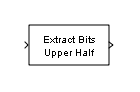# Extract Bits

Output selection of contiguous bits from input signal

• Library:
• Simulink / Logic and Bit Operations

HDL Coder / Logic and Bit Operations

•## Description

The Extract Bits block allows you to output a contiguous selection of bits from the stored integer value of the input signal. Use the Bits to extract parameter to define the method for selecting the output bits.

• Select `Upper half` to output the half of the input bits that contain the most significant bit. If there is an odd number of bits in the input signal, the number of output bits is given by the equation

`number of output bits = ceil(number of input bits/2)`
• Select `Lower half` to output the half of the input bits that contain the least significant bit. If there is an odd number of bits in the input signal, the number of output bits is given by the equation

`number of output bits = ceil(number of input bits/2)`
• Select `Range starting with most significant bit` to output a certain number of the most significant bits of the input signal. Specify the number of most significant bits to output in the Number of bits parameter.

• Select `Range ending with least significant bit` to output a certain number of the least significant bits of the input signal. Specify the number of least significant bits to output in the Number of bits parameter.

• Select `Range of bits` to indicate a series of contiguous bits of the input to output in the Bit indices parameter. You indicate the range in `[start end]` format, and the indices of the input bits are labeled contiguously starting at 0 for the least significant bit.

This block does not report wrap on overflow warnings during simulation. To report these warnings, see the `Simulink.restoreDiagnostic` reference page. The block does report errors due to wrap on overflow.

## Ports

### Input

expand all

Input signal, specified as a scalar, vector, matrix, or N-D array. Floating-point inputs are passed through the block unchanged. `boolean` inputs are treated as `uint8` signals.

Note

Performing bit operations on a signed integer is difficult. You can avoid difficulty by converting the data type of your input signals to unsigned integer types.

Data Types: `single` | `double` | `int8` | `int16` | `int32` | `int64` | `uint8` | `uint16` | `uint32` | `uint64` | `Boolean` | `fixed point`

### Output

expand all

Contiguous selection of extracted bits, specified as a scalar, vector, matrix, or N-D array. Floating-point inputs are passed through the block unchanged.

Data Types: `single` | `double` | `int8` | `int16` | `int32` | `int64` | `uint8` | `uint16` | `uint32` | `uint64` | `fixed point`

## Parameters

expand all

Select the method for extracting bits from the input signal.

Consider an input signal that is represented in binary by `110111001`:

• If you select `Upper half` for the Bits to extract parameter, the output is `11011` in binary.

• If you select `Lower half` for the Bits to extract parameter, the output is `11001` in binary.

• If you select ```Range starting with most significant bit``` for the Bits to extract parameter, and specify `3` for the Number of bits parameter, the output is `110` in binary.

• If you select ```Range ending with least significant bit``` for the Bits to extract parameter, and specify `8` for the Number of bits parameter, the output is `10111001` in binary.

• If you select `Range of bits` for the Bits to extract parameter, and specify `[4 7]` for the Bit indices parameter, the output is `1011` in binary.

#### Programmatic Use

 Block Parameter: `bitsToExtract` Type: character vector Values: ```'Upper half' | 'Lower half' | 'Range starting with most significant bit' | 'Range ending with least significant bit' | 'Range of bits'``` Default: `'Upper half'`

Select the number of bits to output from the input signal. Signed integer data types must have at least two bits. Unsigned data integer types can be as short as a single bit.

#### Dependencies

To enable this parameter, set Bits to extract to `Range starting with most significant bit` or `Range ending with least significant bit`.

#### Programmatic Use

 Block Parameter: `numBits` Type: character vector Values: positive integer Default: `'8'`

Specify a contiguous range of bits of the input signal to output. Specify the range in `[start end]` format. The indices are assigned to the input bits starting with 0 at the least significant bit.

#### Dependencies

To enable this parameter, set Bits to extract to `Range of bits`.

#### Programmatic Use

 Block Parameter: `bitIdxRange` Type: character vector Values: contiguous range Default: `'[0 7]'`

Select the scaling mode to use on the output bit selection:

• When you select ```Preserve fixed-point scaling```, the fixed-point scaling of the input is used to determine the output scaling during the data type conversion.

• When you select ```Treat bit field as an integer```, the fixed-point scaling of the input is ignored, and only the stored integer is used to compute the output data type.

#### Programmatic Use

 Block Parameter: `outScalingMode` Type: character vector Values: ```'Preserve fixed-point scaling' | 'Treat bit field as an integer'``` Default: `'Preserve fixed-point scaling'`

## Block Characteristics

 Data Types `Boolean` | `double` | `fixed point` | `integer` | `single` Direct Feedthrough `yes` Multidimensional Signals `yes` Variable-Size Signals `no` Zero-Crossing Detection `no`

## Version History

Introduced before R2006a